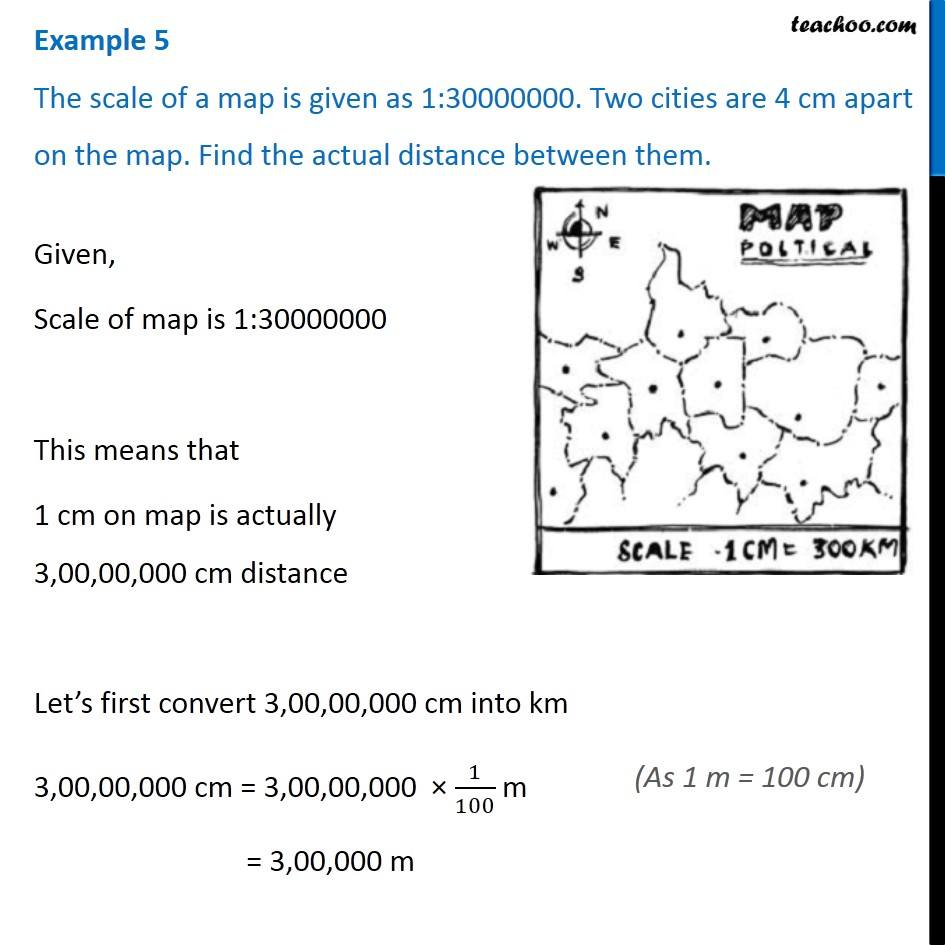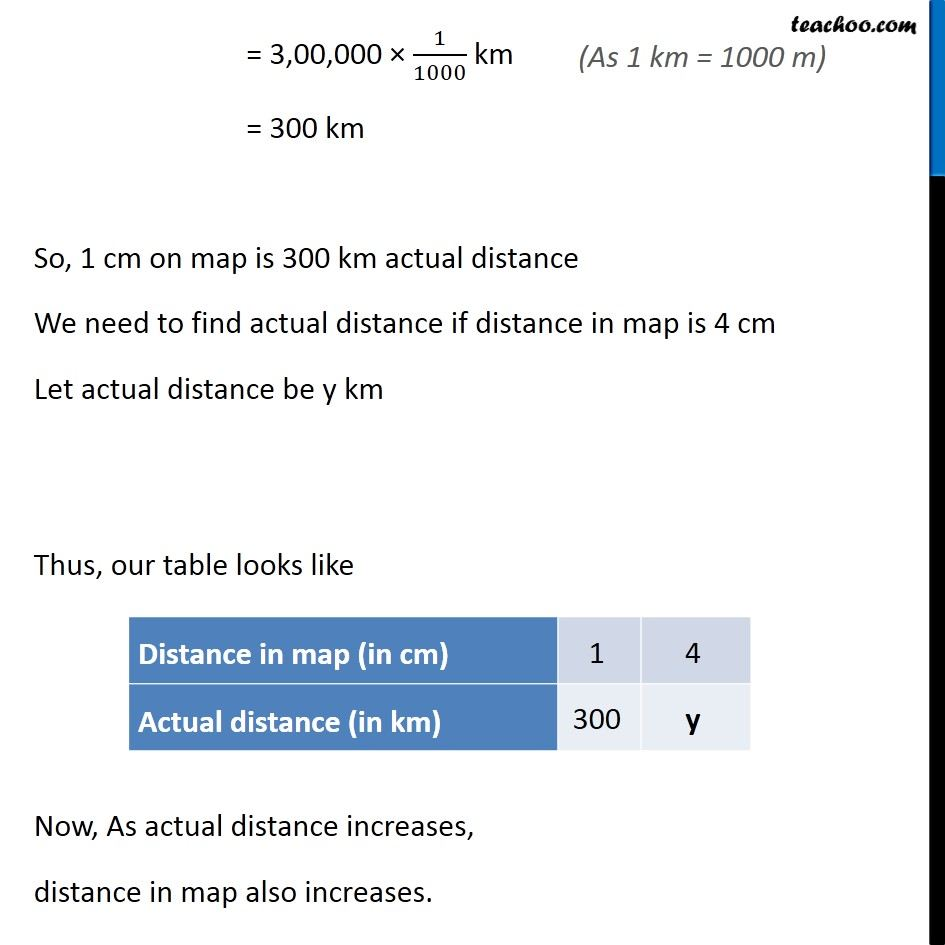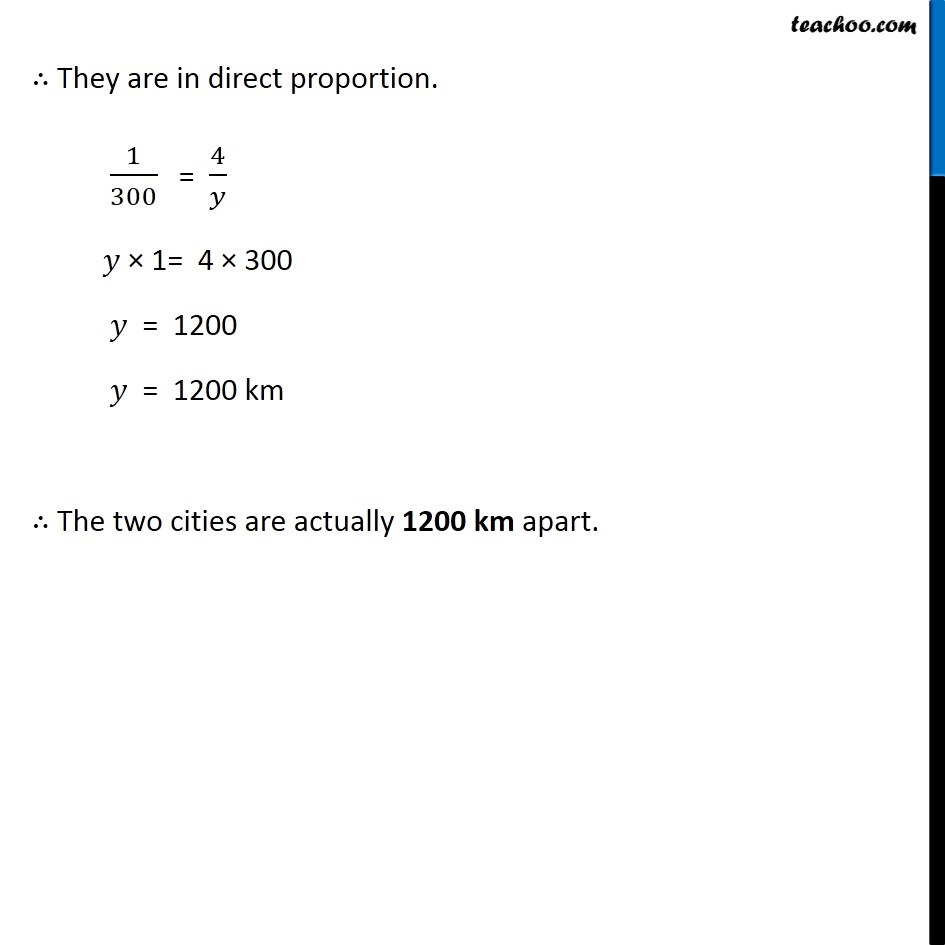Examples

Chapter 13 Class 8 Direct and Inverse Proportions
Serial order wiseGet live Maths 1-on-1 Classs - Class 6 to 12

### Transcript

Example 5 The scale of a map is given as 1:30000000. Two cities are 4 cm apart on the map. Find the actual distance between them. Given, Scale of map is 1:30000000 This means that 1 cm on map is actually 3,00,00,000 cm distance Let’s first convert 3,00,00,000 cm into km 3,00,00,000 cm = 3,00,00,000 × 1/100 m = 3,00,000 m (As 1 m = 100 cm) = 3,00,000 × 1/1000 km = 300 km So, 1 cm on map is 300 km actual distance We need to find actual distance if distance in map is 4 cm Let actual distance be y km Thus, our table looks like Now, As actual distance increases, distance in map also increases. ∴ They are in direct proportion. 1/300 = 4/𝑦 𝑦 × 1= 4 × 300 𝑦 = 1200 𝑦 = 1200 km ∴ The two cities are actually 1200 km apart.